### Instantaneous, Average, Real and Reactive Power

Forward

We discussed and developed some important concepts of transmission lines in last few articles. Last time we discussed about long transmission lines. Here we discuss a simple but important basic concept Electric Power. This will refresh our knowledge before we move further.

Electric Power has same meaning as mechanical power but here the power or energy that we are concerned is in Electrical form. We often encounter terms like instantaneous, average, total, real, reactive, apparent and complex power or simply power. What they mean? how are they related ? That we will discuss here and in next article.

DC Circuit

As long as our analysis is restricted to Direct Current(DC) circuit the power consumed by the resistance load is the product of voltage across the resistance and current flowing through the resistance. It is really simple.

P = V . I
The power consumed by the load is the product of voltage across the load and current drawn by the load (Fig-A). Or the Power supplied by the DC source (battery/cell) is the product of voltage across the cell and current supplied by the cell. Both are equal in our example figure(considering ideal battery of zero internal resistance). The law of energy conservation implies power supplied by the source must be same as power consumed by the circuit. In DC circuit case instantaneous power is same as average power.
AC Circuit

In AC circuit analysis, what is this power that we talk about. The main problem is that the AC voltage and current varies sinusoidally with time. Moreover the presence of circuit reactive elements like Inductor and capacitor shift the current wave with respect to voltage wave (angle of phase difference).

Power is rate at which energy is consumed by load or produced by generator. Whether it is DC circuit or AC circuit, the value of instantaneous power is obtained by multiplying instantaneous voltage with instantaneous current. If at any instant of time t the voltage and current values are represented by sine functions as

v = Vm  sin ωt

i = Im  sin (ωt-φ)

Vm and Im  are the maximum values of the sinusoidal voltage and current. Here ω=2 π f
f is the frequency and ω is the angular frequency of rotating voltage or current phasors. It should be clear that for a power system f is usually 50 or 60 Hz
φ is the phase difference between the voltage and current.

As we said the instantaneous power is the product of instantaneous voltage and current, if we name instantaneous power as p then

p = v.i =  Vm  sin ωt  .  Im  sin (ωt-φ)
or  p = Vm Im  sin ωt  sin (ωt-φ)

Applying trigonometric formula 2.sin A.sin B = cos(A-B) - cos (A+B)  we get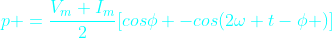It can be written as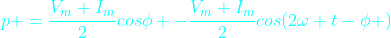This is the equation of instantaneous power

In the Fig-C is drawn all the three waves corresponding to v, i and p. Graphically also we can get the value of instantaneous power (p) at any instant of time t by simply multiplying the value of current i and voltage v at that particular instant t. (You can verify that in the diagram p is negative when either v or i is negative otherwise p is positive. See the points where p is zero). In the graph we have shown horizontal axis as angle φ instead of time t for easy visualization. It should be clear that both way it is correct.

Clearly the instantaneous power p is composed of two terms. The first term is constant because for a given load the phase angle φ is fixed. It does not change unless the load is changed.  The second term  is varying with time sinusoidally due to the presence of the term cos (2ωt-φ). Look that the instantaneous power frequency is twice the frequency of voltage or current.

So the instantaneous power in a single phase circuit varies sinusoidally.

The instantaneous power,  p = constant term + sinusoidal oscillating term.

In one complete period the average of oscillating term is zero.

Then what is the average power within a given time, say one Time Period of the wave?

It is the constant term.

Here is another way to think about the average power.
Just observe that the instantaneous power is negative for a small time. For any time interval you just find the total +ve area A+ (above horizontal-axis (blue line) and below p curve) and total -ve area A- (below horizontal axis and  above p curve). The net area is obtained by subtracting A- from A+. By dividing this net area ( by the time interval T we get the average power(P). You can do this using calculus. What you will ultimately get is only the first term in the above formula for instantaneous power p.

In still another way it is easier to realize that the formula for instantaneous power p has a constant term  (Vm.Im / 2) cos φ and the other sinusoidal term (Vm.Im / 2) cos (2 wt - φ). Actually p is the oscillating power which oscillates about the average constant term  (Vm.Im / 2) cos φ .

So the average power is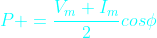The above formula can be written as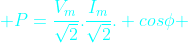Or,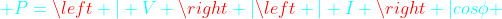here,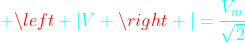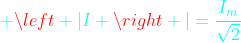V and I are the phasor representation of RMS values* of voltage and current sinusoids. The symbols |V| and |I| are  the magnitudes of phasors V and I. (See at the buttom for definition of RMS value).

This above formula is your favorite formula for useful power that we are most concerned about. This average power formula is used to find the power consumed by the load. The monthly electric energy bill at home is based on this power. The engineers and technicians in power or electrical industry simply use the term power instead of average power. So whenever we simply call power it means average power.

Of course the instantaneous power is oscillating in nature. As we already said it does not oscillates about the horizontal-axis rather about the average power P (cyan color horizontal line).

P will be zero when cos φ =0 or  φ  = 90 degree, that is when the phase angle between voltage and current waves is 90 degrees. It is only when the load is pure inductive or capacitive. In this case the second term only remains in the instantaneous power formula.

From the above figure for some time the power becomes negative that means the load supply energy to source for this period. This is due to the presence of reactive element in load.

The above formula for instantaneous power can be written in another form. This form actually is an attempt to distinguish the oscillating reactive power from the instantaneous power formula.   Rearranging the terms in equation for instantaneous power above we get

p = |V| | I | cos φ (1-cow2ωt) - |V| | I | sin φ sin2ωt

In this equation the first term |V| | I | cos φ (1-cow2ωt) is oscillatory whose average value is |V| | I | cos φ. We already talked about this average power.

The second term |V| | I | sin φ sin2ωt which is also oscillatory but with zero average value. The maximum value of this term is |V| | I | sin φ. This is the so called Reactive power. So Reactive power is the maximum value of a oscillatory power that is repeatedly drawn from the source and again returned to the source within each cycle. So the average of this reactive power is zero.

The average power P is called as Real Power. It is also sometimes called active power.

Real power = P = |V| | I | cos φ

It is usually written as P = VI cos φ. But it should be remembered that V and I are the rms values of voltage and current. For example when we say single phase 220 volt AC it means the rms value of voltage is 220 volts ( it is not maximum value of voltage sinusoid)

Reactive power = Q = |V| | I | sin φ

Real power is measured in Watt and the reactive power is measured in VAR (VoltAmpereReactive). In power sector these units are too small so real power is measured in Megawatt (MW) and reactive power in Megavar (MVAR). The letter R at the end denotes reactive power.

Many times students and practicing engineers are confused about the average power (often simply called power). They think that what they get by multiplying RMS voltage and RMS current is RMS power. No that is wrong. There is no RMS power. RMS power has no meaning or not defined. (Also see definition of RMS value, below at the end). It is average power or real power or true power.

Power In Three phase Balanced System

Let us consider a three phase balanced system. A three phase balanced system is analysed considering only one phase and neutral return. This is called per phase analysis. So the above analysis for single phase is true for balanced three phase case. Let the total power here is Pt. Then we get total three phase power as thrice of single phase case.

P= 3 |V| | I | cos φ

It should be remembered that |V| and | I |  are the per phase values. and φ is the phase angle of load in per phase analysis.

The above formula for balanced three phase system can be written as

P= √3 |Vl| | Il | cos φ

In the above formula Vl and  Il are line voltage and current (Fig-D). This equation is independent of type of three phase load connection i.e delta or star connected load. You have to know the line voltage, line current and phase angle φ as above. This form is very convenient and used often in power calculation.

There is one main difference between the single phase and total three phase power. The instantaneous single phase power is pulsating. In the balanced three phase case, each phase instantaneous power is pulsating but the three pulsating power waves are 120 degrees displaced from each other. At any instant of time the total of these three instantaneous power waves is a constant which is 3 |V| | I | cos φ. So the total power consumed in three phase balanced system is not pulsating. Non-pulsating power also imply the desired non-pulsating torque in case of three phase rotating machines. In large 3-phase motors this is really desired.

*RMS value of AC Sinusoids

The value of AC voltage or current that produces the same heating (or same energy) that is produced if DC voltage or current numerically equal to RMS value of AC is applied instead of AC. This concept helps make the formula for power similar for both DC and AC circuits.

You should read the next article about Power Triangle and Complex Power

hari said...

very clear and this topic which i always confused.thank u very much.

Unknown said...Anonymous said...

I am really happy to read this web site posts which includes tons of
useful facts, thanks for providing such information.
Also see my web page: good ways to make money fastAnonymous said...

Thank you for this site, it is so helpful and explains things so clearlyAnonymous said...

Good work in explaining the concepts crisply. Thank you!

Unknown said...

There is no such thing as Instantaneous Power.

Unknown said...

Nice writeup and easy to understand graphics.Anonymous said...

Amazing. Got it.

Unknown said...

when i calculate instantaneous power phase difference represented two kinds form. (θv - θi) or (θi - θv). what this kinds of phenomenon.
i'm so sorry to my poor english skill.

Unknown said...

explanation in very easy and crucial way....thank you

Unknown said...

explanation in very easy and crucial way....thank you

Allen jeley said...

nic

SabioEremita said...

Good explanation RMS value it's a math definition stipulation, really don't exist in real case, on pure sinusoidal system the active RMS power and average power have much in common, i could show boring calculations proving they are the same, but when you are dealing with non-linear loads? they are in different worlds, but this history is for another time.Anonymous said...

excellent work ....Anonymous said...

Thank you sir..

Unknown said...

thanks good topic delivered

Unknown said...

Good Explanation.

Unknown said...

Ravikumar,

Excellent way of explanation. Thank You

robinjack said...

I am constantly flabbergasted by the measure of data accessible on this subject. What you displayed was very much inquired about and eloquent so as to get your stand on this crosswise over to every one of your perusers. camping generators

Unknown said...

That part about reactive power from instantaneous power is completely dope